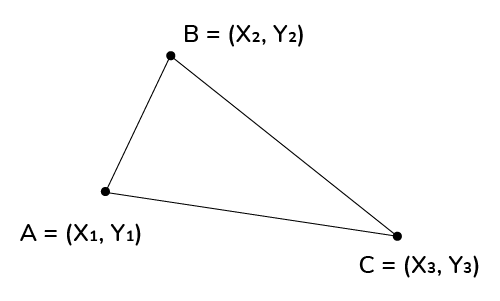# Perimeter of a Triangle With Vertices Calculator

Created by Anna Szczepanek, PhD
Reviewed by Komal Rafay
Last updated: Jun 08, 2022

Omni's perimeter of a triangle with vertices calculator is here for everyone who has ever wondered how to find the perimeter of a triangle with coordinates.

In the article below we will not only give you the formula for the perimeter of a triangle with vertices but also explain why this formula holds so that you'll be able to compute by hand the perimeter of a triangle whose vertices are given if you ever find yourself in such a math emergency. (Under normal circumstances, though, we hope you'll keep using our perimeter of a triangle with vertices calculator!)

## What is the perimeter of a triangle with vertices?

As you surely remember, the perimeter of a triangle is just the distance around its edges. To find the perimeter we need to sum the lengths of our triangle's sides.

So what is the perimeter of a triangle with vertices? This phrase refers to the problem where you don't know the lengths of the triangle's sides, but you only know the coordinates of the triangle's vertices. More calculations are then needed because we have to compute the side lengths from these coordinates. In what follows we'll show you how to do it.

## Formula for the perimeter of triangle with vertices

Finding the perimeter of a triangle with vertices is not complicated, yet requires an intermediate step: we need to compute the length of each side. We do it using the distance formula.

Let's say our vertices are $(x_1, y_1)$, $(x_2, y_2)$, $(x_3, y_3)$. Then the lengths of the sides $AB$, $AC$, $BC$, respectively, read:

\footnotesize \begin{align*} & \textrm{Side } AB = \sqrt{(x_1-x_2)^2+(y_1-y_2)^2} \\ & \textrm{Side } AC = \sqrt{(x_1-x_3)^2+(y_1-y_3)^2} \\ & \textrm{Side } BC = \sqrt{(x_2-x_3)^2+(y_2-y_3)^2} \end{align*}Now we sum the three lengths to determine the perimeter using three vertices:

$\footnotesize P = \textrm{Side } AB + \textrm{Side } AC + \textrm{Side } BC$

That's it! We've just determined the perimeter of a triangle with coordinates.

## How to use this perimeter of a triangle with vertices calculator?

Our tool is really simple to use:

1. Enter the coordinates of the vertices.
2. The perimeter will get calculated immediately.
3. If you need the lengths of sides, click the advanced mode of our perimeter of a triangle with vertices calculator.

## Other triangle perimeter tools

Have you mastered computing the perimeter of a triangle whose vertices are known? We've got more tools related to the perimeter of triangles:

## FAQ

### How do I find the perimeter of a triangle with vertices?

To determine the perimeter using three vertices:

1. Use the distance formula to compute the length of each side of your triangle.
2. Add these three lengths together.
3. The result is exactly the perimeter of your triangle. Congrats!

### What is the perimeter of triangle with vertices?

This phrase means the standard triangle perimeter when we have to compute it using the coordinates of the triangle's vertices via the distance formula (Pythagorean theorem).

Anna Szczepanek, PhDx₁
y₁
x₂
y₂
x₃
y₃
Perimeter
People also viewed…

### Absolute value equation

This absolute value equation calculator will help you to solve equations that involve the absolute value of a linear expression. It shows intermediate calculations and graphs the solutions!

### Black Friday

How to get best deals on Black Friday? The struggle is real, let us help you with this Black Friday calculator!

### Dividing fractions

This dividing fractions calculator can divide up to 5 fractions, in mixed or simple forms.

### Podcasts

Do you feel like you could be doing something more productive or educational while on a bus? Or while cleaning the house? Well, why don't you dive into the rich world of podcasts! With this podcast calculator, we'll work out just how many great interviews or fascinating stories you can go through by reclaiming your 'dead time'!